# GSEB Solutions Class 8 Maths Chapter 1 Rational Numbers InText Questions

Gujarat Board GSEB Textbook Solutions Class 8 Maths Chapter 1 Rational Numbers InText Questions and Answers.

## Gujarat Board Textbook Solutions Class 8 Maths Chapter 1 Rational Numbers InText Questions

Try These (Page 4)

Question 1.
Fill in the blanks in the following table: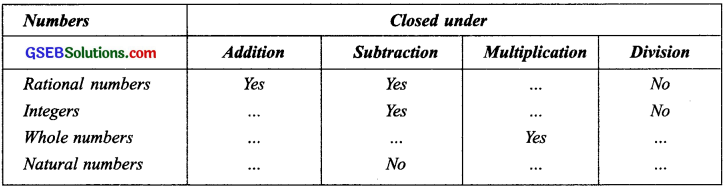Solution:
Using the closure property over addition, subtraction, multiplication and division for rational numbers, integers, whole-numbers and natural numbers, we have: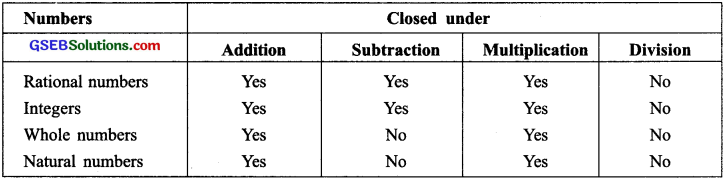Try these (page 6)

Question 1.
Complete the following table: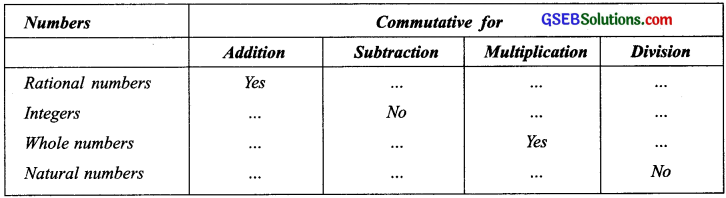Solution: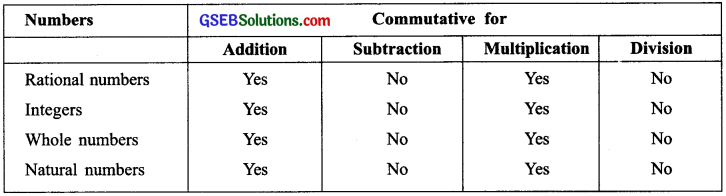Try these (page 9)

Question 1.
Complete the following table: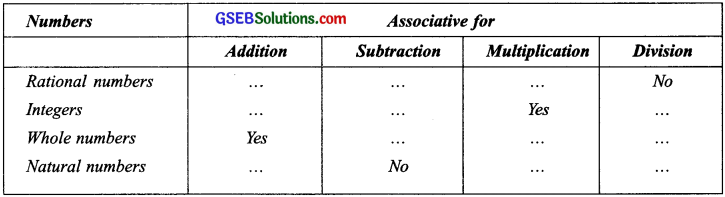Solution: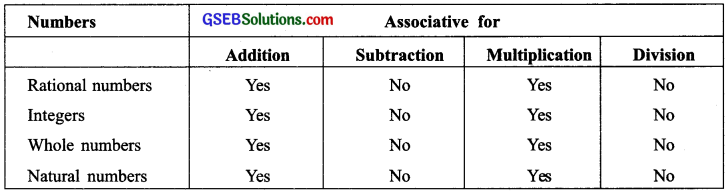Try these (page 11)

Question 1.
If a property holds for rational numbers, will it also hold for integers? For whole numbers? Which will? Which will not?
Solution:

1. Any property which is true for rational numbers, is also true for integers except for any integers ‘a’ and ‘b’, (a + b) is not necessarily an integer.
2. All properties which are true for rational numbers, are also true for whole numbers except:
• For ‘a’ and ‘b’ being whole numbers, (a – b) may not be a whole number.
• For ‘a’ and ‘b’ being whole numbers (b ≠ 0), a + b may not be a whole number.Try these (page 13)

Question 1.
Find using distributivity?
(i) $$\left\{\frac{7}{5} \times\left(\frac{-3}{12}\right)\right\}+\left\{\frac{7}{5} \times \frac{5}{12}\right\}$$

(ii) $$\left\{\frac{9}{16} \times \frac{4}{12}\right\}+\left\{\frac{9}{16} \times \frac{-3}{9}\right\}$$

Solution: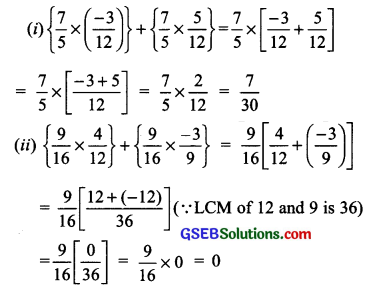Try these (page 17)

Question 1.
Write the rational number for each point labelled with a letter?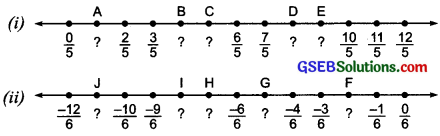Solution:
(i) Here, the rational number for the point A is $$\frac{1}{5}$$
The rational number for the point B is $$\frac{4}{5}$$. The rational number for the point C is $$\frac{5}{5}$$ or 1.
The rational number for the point D is $$\frac{8}{5}$$. The rational number for the point E is $$\frac{9}{5}$$.

(ii) The rational number for:
The point F is $$\frac{-2}{6}$$ or –$$\frac{1}{3}$$. The point G is $$\frac{-5}{6}$$. The point H is $$\frac{-7}{6}$$
The point I is $$\frac{-8}{6}$$ 0r $$\frac{-4}{3}$$. The point J is $$\frac{-11}{6}$$.